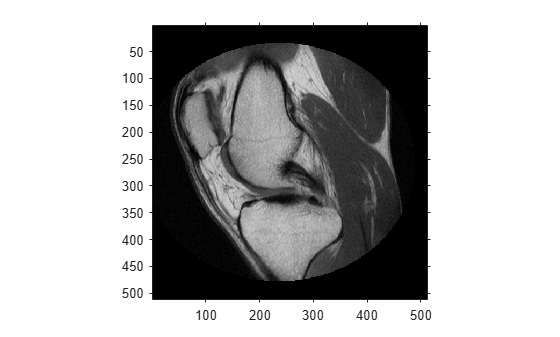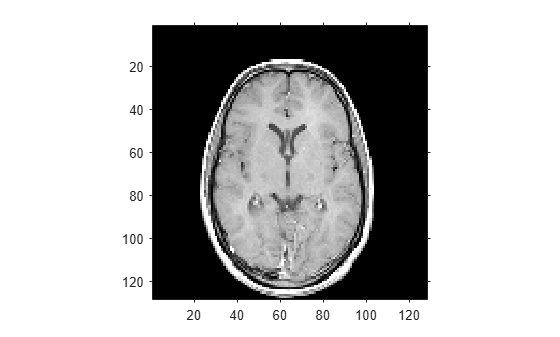intrinsicToWorld

Convert from intrinsic to world coordinates

Description

example

[xWorld, yWorld] = intrinsicToWorld(R,xIntrinsic,yIntrinsic) maps points from the 2-D intrinsic system (xIntrinsic,yIntrinsic) to the 2-D world system (xWorld,yWorld) based on the relationship defined by 2-D spatial referencing object R.

If the kth input coordinates (xIntrinsic(k),yIntrinsic(k)) fall outside the image bounds in the intrinsic coordinate system, intrinsicToWorld extrapolates xWorld(k) and yWorld(k) outside the image bounds in the world coordinate system.

example

[xWorld, yWorld, zWorld] = intrinsicToWorld(R,xIntrinsic,yIntrinsic,zIntrinsic) maps points from the intrinsic coordinate system to the world coordinate system using 3-D spatial referencing object R.

Examples

collapse all

Read a 2-D grayscale image into the workspace.

m = dicominfo('knee1.dcm');

Create an imref2d object, specifying the size and the resolution of the pixels. The DICOM file contains a metadata field PixelSpacing that specifies the image resolution in each dimension in millimeters per pixel.

RA = imref2d(size(A),m.PixelSpacing(2),m.PixelSpacing(1))
RA =
imref2d with properties:

XWorldLimits: [0.1562 160.1562]
YWorldLimits: [0.1562 160.1562]
ImageSize: [512 512]
PixelExtentInWorldX: 0.3125
PixelExtentInWorldY: 0.3125
ImageExtentInWorldX: 160
ImageExtentInWorldY: 160
XIntrinsicLimits: [0.5000 512.5000]
YIntrinsicLimits: [0.5000 512.5000]

Display the image, omitting the spatial referencing object. The axes coordinates reflect the intrinsic coordinates. Notice that the coordinate (0,0) is in the upper left corner.

figure
imshow(A,'DisplayRange',[0 512])
axis onSuppose you want to calculate the approximate position and width of the knee in millimeters. Select the endpoints of a line segment that runs horizontally across the knee at the level of the kneecap. For example, use the (x,y) points (34,172) and (442,172).

xIntrinsic = [34 442];
yIntrinsic = [172 172];

Convert these points from intrinsic coordinates to world coordinates.

[xWorld,yWorld] = intrinsicToWorld(RA,xIntrinsic,yIntrinsic)
xWorld = 1×2

10.6250  138.1250

yWorld = 1×2

53.7500   53.7500

The world coordinates of the two points are (10.625,53.75) and (138.125,53.75), in units of millimeters. The approximate width of the knee in millimeters is:

width = xWorld(2) - xWorld(1)
width = 127.5000

Read a 3-D volume into the workspace. This image consists of 27 frames of 128-by-128 pixel images.

D = squeeze(D);
D = ind2gray(D,map);

Create an imref3d spatial referencing object associated with the volume. For illustrative purposes, provide a pixel resolution in each dimension. The resolution is in millimeters per pixel.

R = imref3d(size(D),2,2,4)
R =
imref3d with properties:

XWorldLimits: [1 257]
YWorldLimits: [1 257]
ZWorldLimits: [2 110]
ImageSize: [128 128 27]
PixelExtentInWorldX: 2
PixelExtentInWorldY: 2
PixelExtentInWorldZ: 4
ImageExtentInWorldX: 256
ImageExtentInWorldY: 256
ImageExtentInWorldZ: 108
XIntrinsicLimits: [0.5000 128.5000]
YIntrinsicLimits: [0.5000 128.5000]
ZIntrinsicLimits: [0.5000 27.5000]

Display the middle slice of the volume, omitting the spatial referencing object. The axes coordinates reflect the intrinsic coordinates. Notice that the coordinate (0,0) is in the upper left corner of this plane. z=0 is right below the first slice, and the z-axis is positive in the upward direction, towards the crown of the head.

figure
imshow(D(:,:,13))
axis onSuppose you want to determine the position, in millimeters, of features within this slice. Select four sample points, and store their intrinsic coordinates in vectors. For example, the first point has intrinsic coordinates (54,46,13). The intrinsic z-coordinate is the same for all points within this slice.

xI = [54 71 57 70];
yI = [46 48 79 80];
zI = [13 13 13 13];

Convert the intrinsic coordinates to world coordinates using intrinsicToWorld.

[xW,yW,zW] = intrinsicToWorld(R,xI,yI,zI)
xW = 1×4

108   142   114   140

yW = 1×4

92    96   158   160

zW = 1×4

52    52    52    52

The resulting vectors are the world x-, y-, and z-coordinates, in millimeters, of the selected points. The first point, for example, is offset from the origin by 108mm in the x-direction, 92 mm in the y-direction, and 52 mm in the z-direction.

Input Arguments

collapse all

Spatial referencing object, specified as an imref2d or imref3d object.

Coordinates along the x-dimension in the intrinsic coordinate system, specified as a numeric scalar or vector.

Data Types: single | double | int8 | int16 | int32 | int64 | uint8 | uint16 | uint32 | uint64

Coordinates along the y-dimension in the intrinsic coordinate system, specified as a numeric scalar or vector. yIntrinsic is the same length as xIntrinsic.

Data Types: single | double | int8 | int16 | int32 | int64 | uint8 | uint16 | uint32 | uint64

Coordinates along the z-dimension in the intrinsic coordinate system, specified as a numeric scalar or vector. zIntrinsic is the same length as xIntrinsic.

Data Types: single | double | int8 | int16 | int32 | int64 | uint8 | uint16 | uint32 | uint64

Output Arguments

collapse all

Coordinates along the x-dimension in the world coordinate system, returned as a numeric scalar or vector. xWorld is the same length as xIntrinsic.

Data Types: single | double | int8 | int16 | int32 | int64 | uint8 | uint16 | uint32 | uint64

Coordinates along the y-dimension in the world coordinate system, returned as a numeric scalar or vector. yWorld is the same length as xIntrinsic.

Data Types: single | double | int8 | int16 | int32 | int64 | uint8 | uint16 | uint32 | uint64

Coordinates along the z-dimension in the world coordinate system, returned as a numeric scalar or vector. zWorld is the same length as xIntrinsic.

Data Types: single | double | int8 | int16 | int32 | int64 | uint8 | uint16 | uint32 | uint64